## 9.4.1 Two-Player Games

To help make the connection to Section 9.3 smoother, two-player games will be considered first. This case is also easier to understand because the notation is simpler. The ideas are then extended without difficulty from two players to many players. The game is formulated as follows.

Formulation 9..8 (A Two-Player Nonzero-Sum Game)
1. The same components as in Formulation 9.7, except the cost function.
2. A function,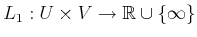, called the cost function for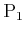.
3. A function,, called the cost function for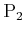.

The only difference with respect to Formulation 9.7 is that now there are two, independent cost functions,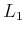and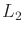, one for each player. Each player would like to minimize its cost. There is no maximization in this problem; that appeared in zero-sum games becausehad opposing interests from. A zero-sum game can be modeled under Formulation 9.7 by setting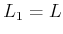and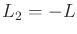.

Paralleling Section 9.3, first consider applying deterministic strategies to solve the game. As before, one possibility is that a player can apply its security strategy. To accomplish this, it does not even need to look at the cost function of the other player. It seems somewhat inappropriate, however, to neglect the consideration of both cost functions when making a decision. In most cases, the security strategy results in regret, which makes it inappropriate for nonzero-sum games.

A strategy that avoids regret will now be given. A pair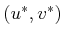of actions is defined to be a Nash equilibrium if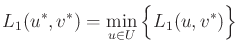(9.66)

and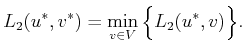(9.67)

These expressions imply that neithernorhas regret. Equation (9.66) indicates thatis satisfied with its action,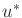, given the action,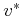, chosen by.cannot reduce its cost any further by changing its action. Likewise, (9.67) indicates thatis satisfied with its action.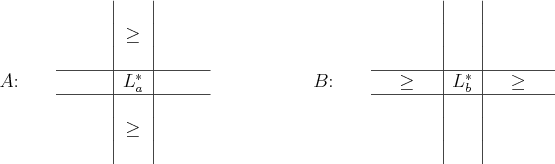The game in Formulation 9.8 can be completely represented using two cost matrices. Let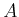and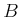denote the cost matrices forand, respectively. Recall that Figure 9.2 showed a pattern for detecting a saddle point. A Nash equilibrium can be detected as shown in Figure 9.5. Think about the relationship between the two. If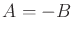, thencan be negated and superimposed on top of. This will yield the pattern in Figure 9.2 (each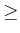becomes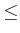because of negation). The values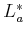and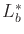coincide in this case. This observation implies that if, then the Nash equilibrium is actually the same concept as a saddle point. It applies, however, to much more general games.

Example 9..17 (A Deterministic Nash Equlibrium)   Consider the game specified by the cost matricesand: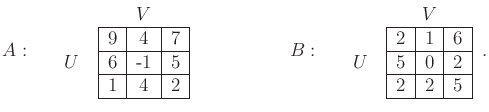(9.68)

By applying (9.66) and (9.67), or by using the patterns in Figure 9.5, it can be seen that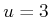and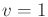is a Nash equilibrium. The resulting costs are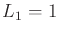and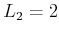. Another Nash equilibrium appears at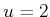and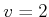. This yields costs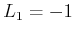and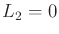, which is better for both players.

For zero-sum games, the existence of multiple saddle points did not cause any problem; however, for nonzero-sum games, there are great troubles. In the example shown here, one Nash equilibrium is clearly better than the other for both players. Therefore, it may seem reasonable that a rational DM would choose the better one. The issue of multiple Nash equilibria will be discussed next.Subsections
Steven M LaValle 2020-08-14